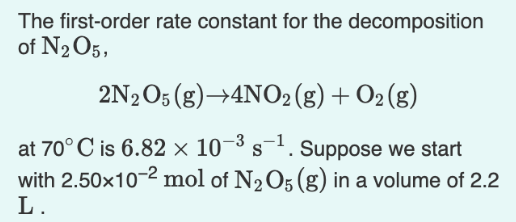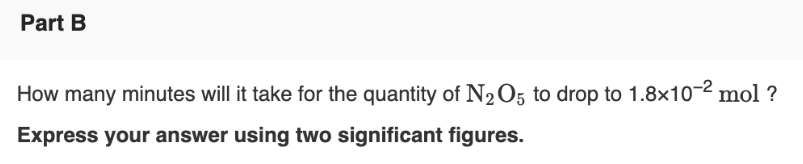# The first-order rate constant for the decomposition of N2O5, 2N2O5 (g) → 4NO2 (g) + O2 (g) at 70 °C is 6.82x10^-3 s^-1. Suppose we start with 2.50x10^-2 mol of N2O5 (g) in a volume of 2.2 L.How many minutes will it take for the quantity of N2O5 to drop to 1.8x10^-2 mol? Express your answer using two significant figures.